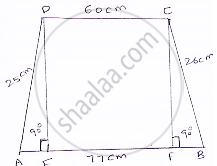# Two Parallel Side of a Trapezium Are 60 Cm and 77 Cm and Other Sides Are 25 Cm and 26 Cm. Find the Area of the Trapezium. - Mathematics

Two parallel side of a trapezium are 60 cm and 77 cm and other sides are 25 cm and 26 cm. Find the area of the trapezium.

#### Solution

Given that two parallel sides of trapezium are AB = 77 and CD = 60 cm
Other sides are BC = 26 m and AD = 25 cm.
Join AE and CF
Now, DE ⊥ AB and CF ⊥ AB
∴ DC = EF = 60 cm
Let AE = x
⇒ BF = 77 – 60 – x = 17 – x
In ΔADE, DE^2 = AD^2 – AE^2 = 25^2 – x^2    [∵ Pythagoras theorem]And in ΔBCF, CF^2= BC^2 – BF^2 [∵ By Pythagoras theorem]

⇒25=sqrt(26^2-(17-x)^2)

⇒25^2-x^2=25^2-(289-x^2-34-x) [ ∵(a-b)^2=a^2-2ab+b^2 ]

⇒265-x^2=676-289-x^2+34x

34x=238

x=7

∴ DE =sqrt(25^2-x^2)=sqrt(625-7^2)=sqrt(516)=24cm

∴ Area of trapezium = 1/2(𝑠𝑢𝑚 𝑜𝑓 𝑝𝑎𝑟𝑎𝑙𝑙𝑒𝑙 𝑠𝑖𝑑𝑒𝑠)×ℎ𝑒𝑖𝑔ℎ𝑡=1/2(60×77)×24=1644cm^2

Concept: Application of Heron’s Formula in Finding Areas of Quadrilaterals
Is there an error in this question or solution?

#### APPEARS IN

RD Sharma Mathematics for Class 9
Chapter 17 Heron’s Formula
Exercise 17.2 | Q 5 | Page 19

Share